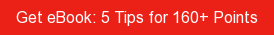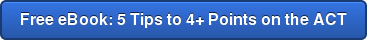# SAT / ACT Prep Online Guides and TipsIf you're going to be taking the AP Biology exam, we have some good news! During the AP test, you'll have access to a formula sheet full of useful equations and definitions. However, you'll need to have a good sense of what's on the AP Bio formula sheet and how to use it in order for it to be useful to you during the test.

That's where we come in! In this guide, we go over everything you need to know about the AP Bio reference sheet. We'll explain every equation and definition it includes, show you what the formula sheet looks like, go over important information it doesn't contain but that you should know, and give you tips on how to get the most out of it.

## What's on the AP Bio Formula Sheet?

The AP Biology formula sheet is actually two pages filled with formulas and definitions that may help you during the AP exam. The formula sheet will be at the beginning of both the multiple-choice and free-response sections of the exam, so you'll have access to it for the entire AP test. That means you don't need to memorize any of the information the formula sheet includes!

So what's on this AP Bio reference sheet? Check it out here, as well as the screenshots below (click on each image to enlarge).

The AP Biology equation sheet covers six main topics. Below the images, we'll list what equations and information each topic includes on the formula sheet.

• Statistical analysis and probability
• Rate and growth
• Surface area and volume
• Water potential
• Dilution
• Gibbs Free Energy

### Statistical Analysis and Probability

Formulas
• Mean
• Standard deviation
• Standard error of the mean (you won't actually calculate using this equation on the exam; it's just for reference)
• Chi-square
• Hardy-Weinberg equations (2)

Information

• Chi-square table
• Laws of probability (2)
• Metric prefixes (8)
• Degrees of freedom explanation
• Definitions for:
• Mode
• Median
• Mean
• Range

### Rate and Growth

Formulas
• Rate
• Population growth
• Exponential growth
• Logistic growth
• Temperature coefficient
• Primary productivity calculation

### Surface Area and Volume

Formulas
• Volume of a sphere
• Volume of a rectangular solid
• Volume of a right cylinder
• Surface area of a sphere
• Surface area of a cube
• Surface area of a rectangular solid

Looking for help studying for your AP exam?

Our one-on-one online AP tutoring services can help you prepare for your AP exams. Get matched with a top tutor who got a high score on the exam you're studying for!### Water Potential

Formulas
• Water potential
• Solute potential of a solution

Information

• Explanation of water potential in an open container

Formulas
• Dilution

### Gibbs Free Energy

Formulas
• Gibbs free energy

### Miscellaneous

Formulas
• pH (you won't be required to perform calculations with this equation on the test)

In all, there are 23 formulas on the AP bio formula sheet. However, as you can see by glancing at the formula sheet, it contains a lot more information than just 23 formulas. That's because the formula sheet includes multiple keys that identify the different symbols used in formulas. This is extremely useful information to have because it gives more context to the formulas. For example, while the water potential section of the formula sheet only contains two formulas, it has a key explaining symbols for pressure potential, solution potential, ionization constant, molar concentration, pressure constant, and temperature in Kelvin.

When you're looking at an equation like, say the one for Gibbs free energy, even if you know (or can see on the formula sheet) that the formula is ΔG = ΔH – TΔS, that doesn't mean much unless you know what each symbol stands for. So in addition to providing you with formulas, the AP Biology equation sheet also gives you the information needed to use those equations more easily.## What Isn't on the AP Bio Formula Sheet That You Need to Know for the Exam?

The AP Biology reference sheet does an excellent job of covering the formulas you need to know for the exam, but there are some other key pieces of information related to the formulas that will be helpful for you to have memorized.

### Population Curves

There are several equations about population growth and change on the AP Biology equation sheet, but you should also know what different population curves look like. There are two main ones to know:

Exponential Growth (J-curve)

Exponential growth is when a population's per capita growth rate remains the same regardless of the population size which causes the population to grow faster as it gets larger. This makes the population growth take the shape of a "J" when graphed. Eventually, this type of rapid population growth will be stopped by a limiting factor in the environment, such as lack of food or space.Logistic Growth (S-curve)

Populations showing logistic growth have their per capita growth shrinking as the population gets closer to the carrying capacity(k), which is the maximum population size possible in the environment. The population levels off once it reaches carrying capacity, due to limits somewhere, such as food, shelter, or space.### pH Rules

There's one lonely pH formula on the AP Bio reference sheet, but pH is an important topic, and there are several other formulas and rules related to it that you should know. Here are three useful pH formulas:

To convert between [H+] and pH: pH = -log[H+] (this equation is included on the formula sheet)

To convert between [OH-] and pOH: pOH = -log[OH-]

For any aqueous solution at 25C: pH + pOH = 14

It's unlikely you'll need to actually do calculations using these formulas, but they're important to know so that you can understand how pH, pOH, [H+], and [OH-], relate to each other. For example, by looking at the first equation above and putting in some sample numbers, you can see that as [H+] gets smaller, pH goes up, and as [H+] gets larger, pH goes down.

You may also be asked if a solution is acidic, basic, or neutral. Here are the rules for that:

• pH of less than 7 means it's acidic
• pH of exactly 7 means it's neutral
• pH of greater than 7 means it's basic## Tips for Getting the Most Out of the AP Bio Formula Sheet

As you can see, the AP Biology equation sheet can be very useful during the exam since it saves you the time of memorizing roughly two dozen equations. In this section we discuss three tips to ensure you get the most out of it.

### #1: Review It, But Don't Memorize It

Some students think that, because they'll have the AP Bio formula sheet with them on exam day, they don't need to worry about it beforehand. However, you don't want to waste precious time during your AP exam hunting through the formulas and trying to figure out which one will be most useful to you. This means you'll need to do some review of the formula sheet well before the test. You should aim to have a general idea of what is included on the formula sheet, as well as where the different equations are. Then, during an exam, you can find the specific formula you're looking for quickly.

### #2: Know How to Use Every Formula

The AP Bio reference sheet contains 23 formulas, and you should know how each of them work before test day. The College Board isn't one for including extraneous information on formula sheets, so if a formula is included on the sheet, that means there's a solid chance you'll need to use it on the exam. Even though you won't use all the formulas on test day, you should know how they all work. You don't want to spend your exam trying to learn how to use, say the Gibbs free energy formula and hoping you got it right!

Well before exam day, be sure to go through every formula on the sheet and make sure you understand it and know how to use it. If you're struggling with one or more formulas, find where it is discussed in your textbook, or ask your teacher for help.

### #3: Take Practice Tests With the Formula Sheet

Answering practice questions and taking practice tests using the AP Bio formula sheet is also key to doing well on the exam. You should take several AP Biology practice tests before the actual exam, and for each of them you should use the official formula sheet. Your teacher will also likely give you a copy of the formula sheet for your in-class exams, so you can get some practice in there too.

If you need help finding practice tests, check out our guide specifically on where to find the best AP Biology practice questions and tests.

## What's Next?

Is AP Biology especially challenging compared to other APs? Read this article for a detailed discussion on the difficulty level of the course and exam.

Want some more guidance preparing for the AP Biology exam? We've created the absolute best AP Biology study guide for you to use, and it has everything you need to ace the test.

Itching to get started with AP Bio prep right away? We cover cell theoryenzymes, cell structures (endoplasmic reticulum and cell membrane), homologous and analogous structures, and the photosynthesis equation with subject-specific guides.

Want to improve your SAT score by 160 points or your ACT score by 4 points? We've written a guide for each test about the top 5 strategies you must be using to have a shot at improving your score. Download it for free now:Christine Sarikas

Christine graduated from Michigan State University with degrees in Environmental Biology and Geography and received her Master's from Duke University. In high school she scored in the 99th percentile on the SAT and was named a National Merit Finalist. She has taught English and biology in several countries.

Student and Parent Forum

Our new student and parent forum, at ExpertHub.PrepScholar.com, allow you to interact with your peers and the PrepScholar staff. See how other students and parents are navigating high school, college, and the college admissions process. Ask questions; get answers.